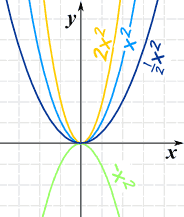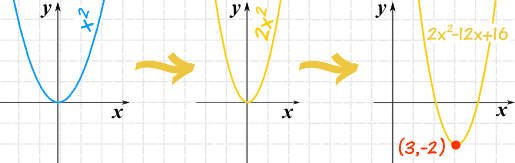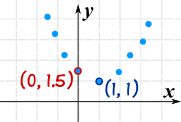A Quadratic Equation in Standard Form
(a, b, and c can have any value, except that a can't be 0.)

Here is an example:## Graphing

You can graph a Quadratic Equation using the Function Grapher, but to really understand what is going on, you can make the graph yourself. Read On!

f(x) = x2

And its graph is simple too:This is the curve f(x) = x2
It is a parabola.

Now let us see what happens when we introduce the "a" value:

f(x) = ax2• Larger values of a squash the curve inwards
• Smaller values of a expand it outwards
• And negative values of a flip it upside down## Play With It

Now is a good time to play with the
"Quadratic Equation Explorer" so you can
see what different values of a, b and c do.

Before graphing we rearrange the equation, from this:

f(x) = ax2 + bx + c

To this:

f(x) = a(x-h)2 + k

Where:

• h = −b/2a
• k = f( h )

In other words, calculate h (= −b/2a), then find k by calculating the whole equation for x=h

## But Why?The wonderful thing about this new form is that h and k show us the very lowest (or very highest) point, called the vertex:

And also the curve is symmetrical (mirror image) about the axis that passes through x=h, making it easy to graph

### So ...

• h shows us how far left (or right) the curve has been shifted from x=0
• k shows us how far up (or down) the curve has been shifted from y=0

Lets see an example of how to do this:

### Example: Plot f(x) = 2x2 − 12x + 16

First, let's note down:

• a = 2,
• b = −12, and
• c = 16

Now, what do we know?

• a is positive, so it is an "upwards" graph ("U" shaped)
• a is 2, so it is a little "squashed" compared to the x2 graph

Next, let's calculate h:

h = −b/2a = −(−12)/(2x2) = 3

And next we can calculate k (using h=3):

k = f(3) = 2(3)2 − 12·3 + 16 = 18−36+16 = −2

So now we can plot the graph (with real understanding!):We also know: the vertex is (3,−2), and the axis is x=3

## From A Graph to The Equation

What if we have a graph, and want to find an equation?

### Example: you have just plotted some interesting data, and it looks Quadratic:Just knowing those two points we can come up with an equation.

Firstly, we know h and k (at the vertex):

(h, k) = (1, 1)

So let's put that into this form of the equation:

f(x) = a(x-h)2 + k

f(x) = a(x−1)2 + 1

Then we calculate "a":

We know the point (0, 1.5) so:f(0) = 1.5
And a(x−1)2 + 1 at x=0 is:f(0) = a(0−1)2 + 1
They are both f(0) so make them equal: a(0−1)2 + 1 = 1.5
Simplify:a + 1 = 1.5
a = 0.5

And so here is the resulting Quadratic Equation:

f(x) = 0.5(x−1)2 + 1

Note: This may not be the correct equation for the data, but it’s a good model and the best we can come up with.